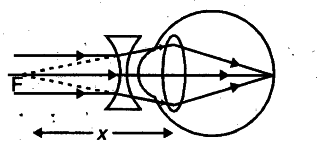# A person suffering from an eye-defect uses lenses of power -1D

A person suffering from an eye-defect uses lenses of power -1D. Identify the eye defect. Draw a suitable diagram to illustrate how this type of lens corrects the defect. Give the nature of corrective lens to be used. Also, calculate the focal length of corrective lens.

The person is suffering from short sightedness. The diagram is as shown :A concave lens is used for this defect.
We know that P = l/f(in m) or f = 1/P = 1/-1 = -1 m = -100 cm